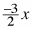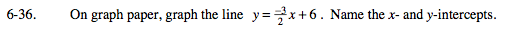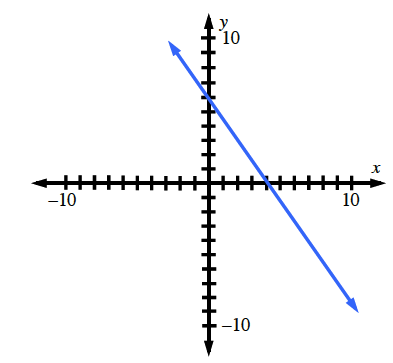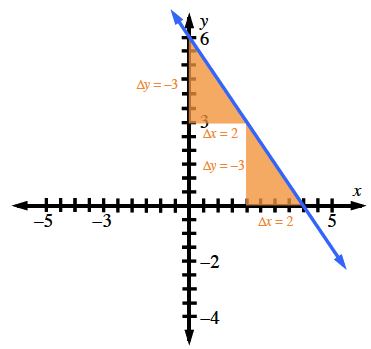Home > GC > Chapter 6 > Lesson 6.1.4 > Problem6-36

6-36.

On graph paper, graph the line y =+ 6 . Name the x­ and y-­intercepts. Homework Help ✎$\text{slope}=\frac{\Delta y}{\Delta x}=-\frac{3}{2}$

A good place to start: (0,6).

Look at the graph. Where does the line cross the x-axis, and where does it cross the y-axis?